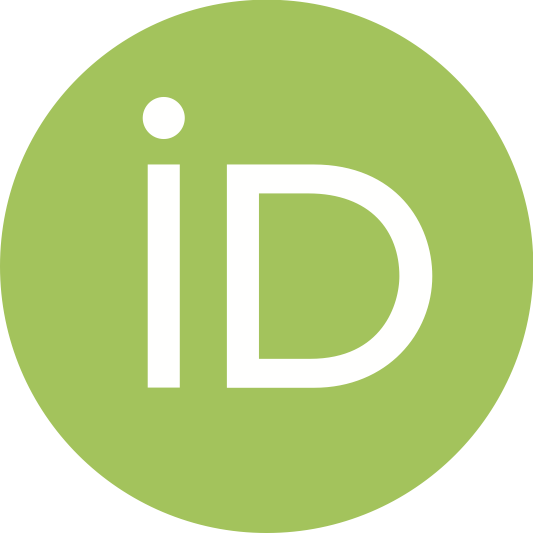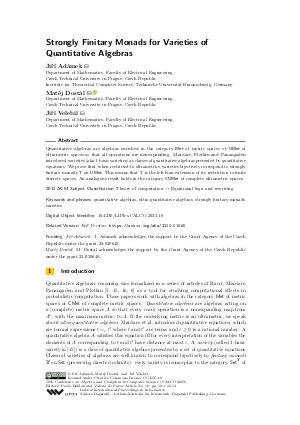Document# Strongly Finitary Monads for Varieties of Quantitative Algebras

### Authors Jiří Adámek, Matěj Dostál, Jiří Velebil## File

LIPIcs.CALCO.2023.10.pdf
• Filesize: 0.75 MB
• 14 pages

## Cite As

Jiří Adámek, Matěj Dostál, and Jiří Velebil. Strongly Finitary Monads for Varieties of Quantitative Algebras. In 10th Conference on Algebra and Coalgebra in Computer Science (CALCO 2023). Leibniz International Proceedings in Informatics (LIPIcs), Volume 270, pp. 10:1-10:14, Schloss Dagstuhl - Leibniz-Zentrum für Informatik (2023)
https://doi.org/10.4230/LIPIcs.CALCO.2023.10

## Abstract

Quantitative algebras are algebras enriched in the category Met of metric spaces or UMet of ultrametric spaces so that all operations are nonexpanding. Mardare, Plotkin and Panangaden introduced varieties (aka 1-basic varieties) as classes of quantitative algebras presented by quantitative equations. We prove that, when restricted to ultrametrics, varieties bijectively correspond to strongly finitary monads T on UMet. This means that T is the left Kan extension of its restriction to finite discrete spaces. An analogous result holds in the category CUMet of complete ultrametric spaces.

## Subject Classification

##### ACM Subject Classification
• Theory of computation → Equational logic and rewriting
##### Keywords
• quantitative algebras
• ultra-quantitative algebras
• varieties

## Metrics

• Access Statistics
• Total Accesses (updated on a weekly basis)
0

## References

1. J. Adámek. Varieties of quantitative algebras and their monads. In Proceedings of the 37th Annual Symposium on Logic in Computer Science (LICS 2022), pages 1-12, 2022.2. J. Adámek, M. Dostál, and J. Velebil. A categorical view of varieties of ordered algebras. Math. Struct. Comput. Sci., 32(4):349-373, 2022.3. J. Adámek, M. Dostál, and J. Velebil. Quantitative algebras and a classification of metric monads, 2023. arXiv:2210.01565.4. J. Adámek and J. Rosický. Approximate injectivity and smallness in metric-enriched categories. J. Pure Appl. Algebra, 226:1-30, 2022.5. G. Bacci, R. Mardare, P. Panaganden, and G. D. Plotkin. An algebraic theory of Markov processes. In Proceedings of Logic in Computer Science (LICS 2018), pages 679-688. ACM, 2018.6. G. Bacci, R. Mardare, P. Panaganden, and G. D. Plotkin. Tensors of quantitative equational theories. In Proceedings of Coalgebraic and Algebraic Methods in Computer Science (CALCO 2021), 2021.7. M. Barr and Ch. Wells. Toposes, triples and theories. Springer-Verlag, New York, 1985.8. F. Borceux. Handbook of Categorical Algebra: Volume 2, Categories and Structures. Cambridge Univ. Press, 1994.9. J. Bourke and R. Garner. Monads and theories. Adv. Math., 351:1024-1071, 2019.10. E. J. Dubuc. Kan Extensions in Enriched Category Theory, volume 145 of Lecture Notes in Mathematics. Springer-Verlag, 1970.11. G. M. Kelly. Basic concepts of enriched category theory. Number 64 in London Math. Soc. Lecture Notes Series. Cambridge Univ. Press, 1982. Also available as Repr. Theory Appl. Categ. 10 (2005).12. G. M. Kelly and S. Lack. Finite-product-preserving functors, Kan extensions and strongly-finitary 2-monads. Appl. Categ. Structures, 1:85-94, 1993.13. A. Kurz and J. Velebil. Quasivarieties and varieties of ordered algebras: regularity and exactness. Math. Structures Comput. Sci., pages 1-42, 2016.14. S. Mac Lane. Categories for the working mathematician. Springer, 2nd edition, 1998.15. R. Mardare, P. Panangaden, and G. D. Plotkin. Quantitative algebraic reasoning. In Proceedings of Logic in Computer Science (LICS 2016), pages 700-709. IEEE Computer Science, 2016.16. R. Mardare, P. Panangaden, and G. D. Plotkin. On the axiomatizability of quantitative algebras. In Proceedings of Logic in Computer Science (LICS 2017), pages 1-12. IEEE Computer Science, 2017.17. M. Mio, R. Sarkis, and V. Vignudelli. Beyond nonexpansive operations in quantitative algebraic reasoning. In Proceedings of Logic in Computer Science (LICS 2022), pages 1-13, 2022. Article 52.X

Feedback for Dagstuhl Publishing